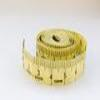#### You may also like### Bigger or Smaller?

When you change the units, do the numbers get bigger or smaller?

##### Age 14 to 16Challenge Level

Some units are particularly well suited to a given measurement because they turn out to give a small, whole number of units. Understanding this concept gives you a feel for the order of magnitude of the quantities.

What units would you measure these quantities in to make an approximation which was a reasonably small whole number?

• The volume of a bacterium
• The mass of an oak tree
• The area of a leaf
• The half-life of Uranium-235
• The area of a football pitch
• The average lifespan of a human
• The volume of a bath tub
Can you reverse the process? For the following units, what physical phenomena might naturally be measured to be around 1 unit?

• Millimetre
• Newton
• Angstrom
• Joule
• Volt
• Microgram
• Micrometre
• Hertz
• Parsec